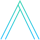# What is a DAG?

May 06, 2019

A graph is a data structure, defined by its vertices and edges. Oftentimes you’ll see it defined as G = (V, E). That just means, “G is the graph defined by vertices V and edges E”.

We usually draw them like this:We call a graph connected if there is a path from any vertex to any other vertex.A cycle in a graph is a path from a vertex back to itself, where each edge is only travelled once (the above graph does not contain a cycle).In a directed graph, each edge has a direction, meaning it goes from one vertex to another vertex. We draw directed graphs like this.Directed graphs can have cycles too, like the above one in red. When a directed graph doesn’t have a cycle, we call it a directed acyclic graph (a DAG). (The changed edge to remove the cycle is shown in gold).In short, that is a directed acyclic graph. A graph that is directed and has no cycles.

# .css-16kqvob{margin-left:-20px;padding-right:4px;color:#212121;}And what is a tree?

A related but different concept is a tree. A tree (in graph theory) is a graph where there is exactly one path from any vertex to any other. Or, more formally, a connected, undirected, acyclic graph.Think of a family tree.

We’ll dive deeper into the DAG in articles to come.